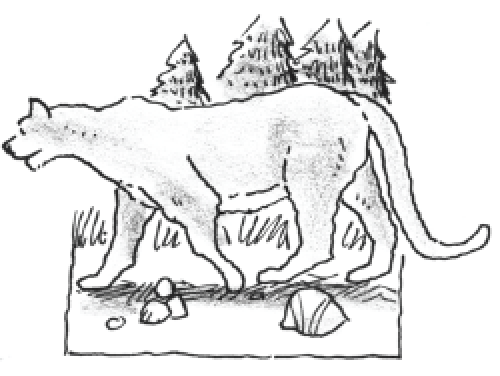### Home > APCALC > Chapter 11 > Lesson 11.3.2 > Problem11-99

11-99.

The population $P$ of mountain lions grows at a rate of  $\frac { d P } { d t }=\frac { 1 } { 160 }P(40 − P)$ lions per year. At time $t = 0$, there are $12$ lions. What is the population after three years?

$\frac{160dP}{P(40-P)}=dt$

Partial fraction decomposition needs to be used.

$\frac{A}{P}+\frac{B}{40-P}=\frac{1}{P(40-P)}$

$160\int\Big(\frac{A}{P}+\frac{B}{40-P}\Big)dP=\int dt$

$160(A\ln|P|-B\ln|40-P|)=t+C$

Solve for $C$ when $t = 0$ and $P = 12$.

Substitute your value for $C$ into the equation from Step 4 along with $t = 3$. Then solve for $P$.